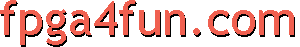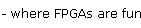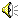## Music box 1 - Simple beep

FPGAs can easily implement binary counters. For example, a 16bit counter is going to count from 0 to 65535 (65536 different values) before rolling-back. The Pluto board has a 25MHz clock oscillator, so we can easily build a 25MHz clocked 16bit free running counter. Its highest bit toggles at a frequency of 25000000/65536=381Hz.

The Verilog HDL code looks like this:(click above on the speaker icon to listen to the result)

 module music(clk, speaker); input clk; output speaker; // first create a 16bit binary counter reg [15:0] counter; always @(posedge clk) counter <= counter+1; // and use the most significant bit (MSB) of the counter to drive the speaker assign speaker = counter; endmodule

In more details, "clk" runs at 25MHz, "counter" looks like a 12.5MHz signal (it is updated at 25MHz with alternate values 0 1 0 1... so it looks like a 12.5MHz signal), "counter" a 6.125MHz signal, and so on.
Since we use the MSB (bit 15) of the counter to drive the output, a nice 381Hz square signal comes out of the "speaker" output.
##### "A" note (440Hz)
Ok, better than a random frequency, why not try to get a 440Hz signal. That's the frequency of the "A" note.

Instead of dividing 25MHz by 65536, we need to divide by 56818.

 module music(clk, speaker); input clk; output speaker; reg [15:0] counter; always @(posedge clk) if(counter==56817) counter <= 0; else counter <= counter+1; assign speaker = counter; endmodule

There is a problem with our implementation though. The frequency is 440Hz, as expected, but the output duty cycle is not 50% anymore. The low level goes from counter=0 to counter=32767 (when bit 15 of counter is low) and then high level from 32768 to 56817. That gives us "speaker" being high only 42% of the time. The easiest way to get a 50% duty cycle is to add a stage that divides the output by 2. So first we divide by 28409 (instead of 56818) and then by 2.

 module music(clk, speaker); input clk; output speaker; reg [14:0] counter; always @(posedge clk) if(counter==28408) counter<=0; else counter <= counter+1; reg speaker; always @(posedge clk) if(counter==28408) speaker <= ~speaker; endmodule
##### Adding a parameter
Here's essentially the same code. A new parameter named "clkdivider" was added, and the counter was changed into a "count-down" counter - just a matter of preference.

 module music(clk, speaker); input clk; output speaker; parameter clkdivider = 25000000/440/2; reg [14:0] counter; always @(posedge clk) if(counter==0) counter <= clkdivider-1; else counter <= counter-1; reg speaker; always @(posedge clk) if(counter==0) speaker <= ~speaker; endmodule